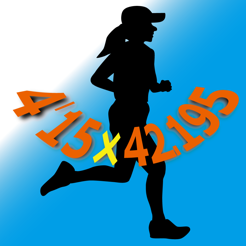## iPhone Screenshots

•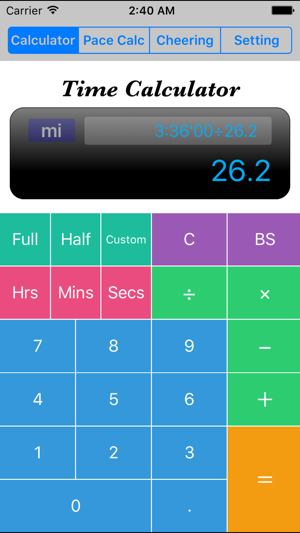•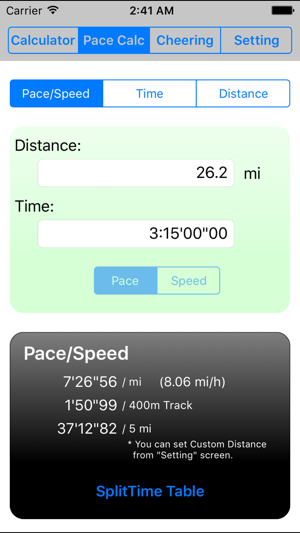•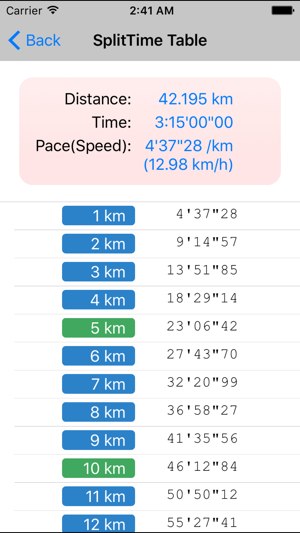•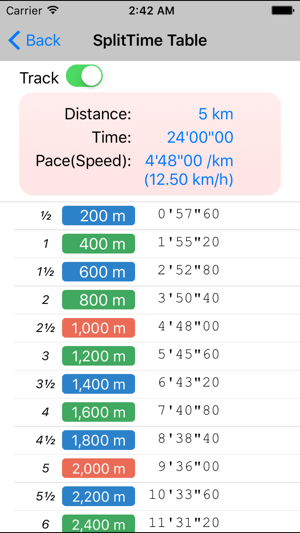•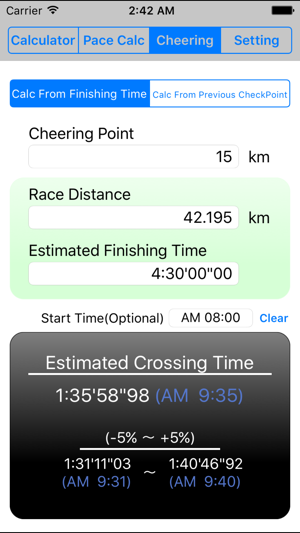## Description

This is a calculator that allows all runners to simply calculate their lap-time and pace.
It feels the same to use as a typical calculator, but it can also carry out for different operations for calculating time.
Furthermore, it also has a special screen used to calculate a runner's pace prepared below.

- Judging by the distance(km or miles) traveled and time elapsed, the calculator can determine the pace and speed of a runner.
- Judging by the pace (or speed) and distance(km or miles) traveled by a runner, the calculator can determine the time elapsed.
- Judging by the pace (or speed) and time elapsed, the calculator can determine the distance(km or miles) traveled.

----- Ver2.2 Update -----

- Track mode has now been added to split time tables
On occasions where distance equals 800m(0.8km)/1000m(1km)/1500m(1.5km)/2000m(2km)/5000m(5km)/10000m(10km), split time tables can now be displayed on the basis of track distance, instead of 1km intervals.

- A search function has been added for crossing times at cheering points
A mode has been added to estimate cheer point crossing times, calculated from estimated finish times or crossing times for the preceding lap point.
Calculate from estimated finishing time / Calculate from crossing time for preceding checkpoint

----- Ver2.0 Update -----

The calculator is compatible with four inch displays and the iOS7 screen.

The user can change the settings from full-marathon, half-marathon, or a user-determined distance with only one touch.

The user can insert seconds, minutes or hours into the time display.

The pace calculating screen can also be used to show split-times.

Using the backspace button, the user can return to their entries prior to their current one.

----- Calculation Rule -----

Measuring time requires choosing seconds, minutes or hours as the lowest unit of calculation.

The numerical value of time for addition will be calculated using seconds.

In the case that numerical values are subtracted from time, calculations will be done using seconds.

Numerical values cannot be subtracted from time.

When the numerical value is divided by time, speed is calculated.

The multiplication of numbers and time will become time.
However, in the case that the previous calculation's result was speed, the numerical value of distance shall be calculated.

Time cannot be multiplied by Time.

## What’s New

Version 2.2.0

・Track mode has now been added to split time tables
On occasions where distance equals 800m(0.8km)/1000m(1km)/1500m(1.5km)/2000m(2km)/3000m(3km)/5000m(5km)/10000m(10km), split time tables can now be displayed on the basis of track distance, instead of 1km intervals.

・A search function has been added for crossing times at cheering points
A mode has been added to estimate cheer point crossing times, calculated from estimated finish times or crossing times for the preceding lap point.

## Ratings and Reviews

4.0 out of 5
4 Ratings

4 Ratings

Lean'n'mean ,

### Excellent and very useful tool for running pace / distance calculations

Very handy for training; thoughtful. Love the time calculator!

## Information

Seller
Makoto Hasizume
Size
1.4 MB
Compatibility

Requires iOS 8.0 or later. Compatible with iPhone, iPad, and iPod touch.

Languages

English, Japanese

Age Rating
4+
•